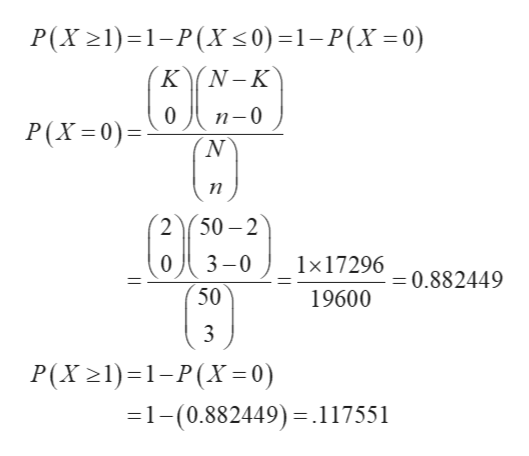# In a group of 50 ballpoint pens on a shelf in the stationary department of Metro Department Store, 2 are known to be defective. (Round your answers to three decimal places.)(a) If a customer selects 3 of these pens, what is the probability that at least 1 is defective?(b) If a customer selects 3 of these pens, what is the probability that no more than 1 is defective?

Question
24 views

In a group of 50 ballpoint pens on a shelf in the stationary department of Metro Department Store, 2 are known to be defective. (Round your answers to three decimal places.)

(a) If a customer selects 3 of these pens, what is the probability that at least 1 is defective?

(b) If a customer selects 3 of these pens, what is the probability that no more than 1 is defective?
check_circle

Step 1

(a)

There are total 50 pens so, N = 50 out if 50, 2 pens are defective and else are not defective.

Let X be the number of pens that are defective in randomly selected n ...help_outlineImage TranscriptioncloseP(X21)1-P(X <0)=1-P(X=0) KN-K 0 п-0 P(X 0) п 50-2 3-0 1x17296 =0.882449 50 19600 P(X 21) 1-P(X= 0) 1-(0.882449).117551 fullscreen

### Want to see the full answer?

See Solution

#### Want to see this answer and more?

Solutions are written by subject experts who are available 24/7. Questions are typically answered within 1 hour.*

See Solution
*Response times may vary by subject and question.
Tagged in

### Math